TUTORIALS
TUTORIALS HOME

GENERAL MATH
NUMBER SETS
ABSOLUTE VALUE & INEQUALITIES
SETS & INTERVALS
FRACTIONS
POLYNOMIALS
LINEAR EQUATIONS
GEOMETRY
FINITE SERIES
TRIGONOMETRY
EXPONENTS
LOGARITHMS
INDUCTION

CALCULUS
LIMITS
DERIVATIVES
RELATED RATES & OPTIMIZATION
CURVE SKETCHING
INTEGRALS
AREA & VOLUME
INVERSE FUNCTIONS

MAIN
HOME
TESTS
TUTORIALS
SAMPLE PROBLEMS
COMMON MISTAKES
STUDY TIPS
GLOSSARY
CALCULUS APPLICATIONS
MATH HUMOUR

# Angles

The classical concept of trigonometry deals with the relationships between the angles and sides of triangles. Over time, however, trigonometry has been adapted so that the angles do not necessarily represent angles in a triangle. For example, in calculus, trigonometric functions are defined for arbitrary real numbers.

Angles can be expressed in degrees or radians. To convert a measurement from radians to degrees (or vice versa) we use the following relationship: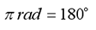This relationship gives the following two equations: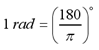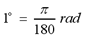Note: By convention, most angles are expressed in radian measure, unless otherwise stated.

# Examples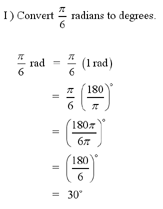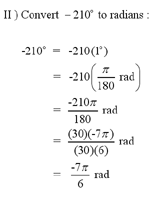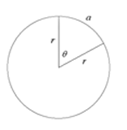# Arc Length

The arc length formula defines the relationship between arc length a, radius r and the angle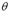(in radians).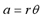Note: Make sure that your angles are measured in radians. The arc length formula does not hold for angles measured in degrees. Use the conversion relationship above to convert your angles from degrees to radians.

Note: For an example of an arc length question, see question #1 in the Additional Examples section at the bottom of the page.

# Trigonometric Functions

The following ratios are for right angle trigonometry. The angle must be acute (angle is less than 90°).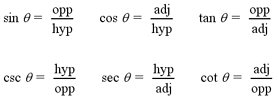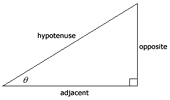For angles that are obtuse (angle is greater than 90°) or negative, we use the following trigonometric ratios. The x and y variables are the values of the x and y coordinates, respectively. The r variable represents the distance from the origin, to the point (x,y). This value can be found using the Pythagorean theorem.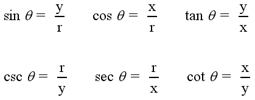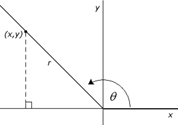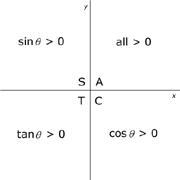When negative or obtuse angles are used in trigonometric functions, they will sometimes produce negative values. The CAST graph to the left will help you to remember the signs of trigonometric functions for different angles. The functions will be negative in all quadrants except those that indicate that the function is positive. For example, When the angle is between 0° and 90° (0 and pi/2 radians), the line r is in the A quadrant. All functions will be positive in this region. When the angle is between 90° and 180° (pi/2 and pi radians), the line is in the S quadrant. This means that only the sine function is positive. All other functions will be negative.

Note: For examples of finding trigonometric ratios see questions #2 and #3 in Additional Examples at the bottom of the page.

# Graphs of Trigonometric Functions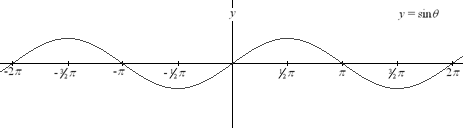Sine Function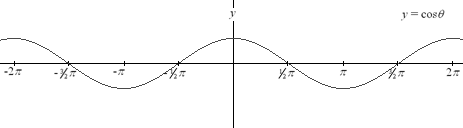Cosine Function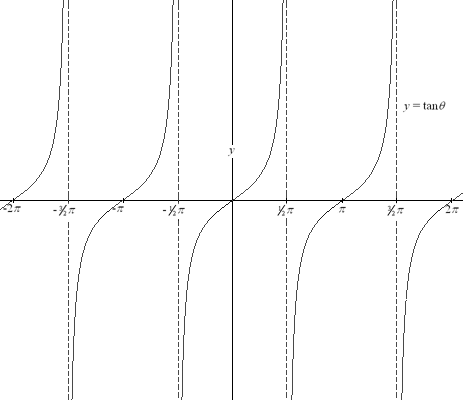Tangent Function

Note: As illustrated in the graphs above, the sine and cosine functions are defined for all values of x. The tangent function, however, being equal to sin x / cos x, is undefined whenever cos x = 0.

# Periodicity of Trigonometric Function

From the graphs above, you can see that trigonometric functions are periodic. The sine and cosine functions, for example, have a period of 2 pi. In general, for any integer k,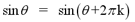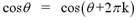The tangent function, however, has a period of pi. The period of the tangent function is given by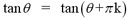for any integer k.

This allows us to easily graph trigonometric functions. We must only determine the graph of the function over its period.

Examples of periodicity can be easily shown using a calculator. For example, if you were to solve sin(160pi) using your calculator, you would find that it is equal to sin(0)=0. This is because sin(160pi) = sin(0 + 2pi(80)).

Using this concept of periodicity, we can calculate certain trigonometric functions without using our calculators. For example, tan(31pi/3) is equal to tan(pi/3 + pi(10)), or equivalently tan(pi/3). From the table in the section below, we know that tan(pi/3) is equal to the square root of 3. So, tan(31pi/3) is also equal to root 3.

# Special Triangles

 I)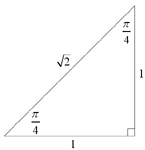II)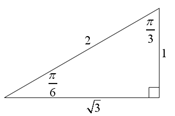Using the "special" triangles above, we can find the exact trigonometric ratios for angles of pi/3, pi/4 and pi/6. These triangles can be constructed quite easily and provide a simple way of remembering the trigonometric ratios. The table below lists some of the more common angles (in both radians and degrees) and their exact trigonometric ratios.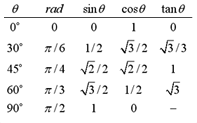# The Laws of Sines and Cosines

The laws of sines and cosines are useful in determining the sides and angles of oblique triangles. All triangles that are not right angled are classified as oblique triangles. The oblique triangle below will be used in the definitions for the laws of sines and cosines.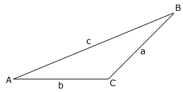The law of sines states: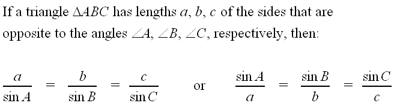Note: All the sides and angles of a triangle can be determined using the law of sines if you know the measurements of any 2 angles and any one side.

Note: For an example of solving a triangle using the sine law, see question #4 in the Additional Examples section at the bottom of the page.

The law of cosines states: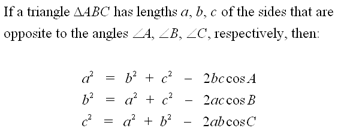Note: All sides and angles of a triangle can be determined using the law of cosines if you know the measurements of 2 sides and the angle enclosed between them, or the lengths of all 3 sides.

Note: For an example of solving a triangle using the cosine law, see question #5 in the Additional Examples section at the bottom of the page.

• | Proof of the cosine law

# Trigonometric Identities

The identities listed below are the basic trigonometric identities. They can be combined with one another to create many more identities.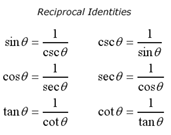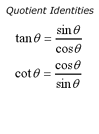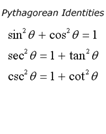# Trigonometric Formulas

The trigonometric formulas below can be combined with the identities above to create very complex trigonometric identities. These formulas are often necessary when proving trigonometric identities.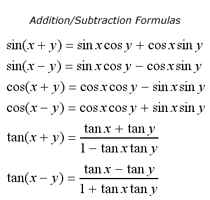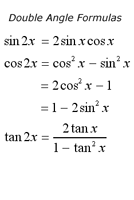Note: For an example of proving a trigonometric identity, see question #6 in the Additional Examples section below.

1 | Find the angle, given arc length and radius
2 | Find all trigonometric ratios of the triangle
3 | Find the exact trigonometric ratios for a given angle
4 | Solve the triangle using the law of sines
5 | Solve the triangle using the law of cosines
6 | Prove the trigonometric identity

| Top of Page |

COURSE HOMEPAGES
MATH 1036
MATH 1037

FACULTY HOMEPAGES
Alex Karassev
Ted Chase